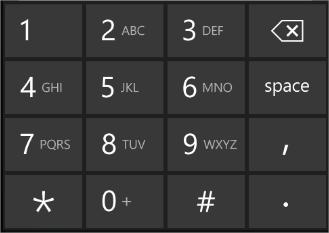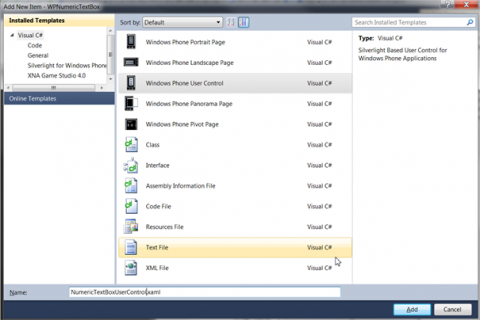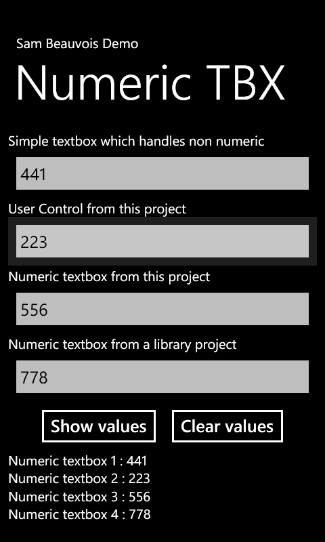Windows Phone 7 Numeric TextBox

There is no â€œbuilt inâ€ numeric textbox in the current Silverlight for Windows phone.

Here a few solutions to realize one anyway : it remembers me the early years of the .NET framework or nothing was in the framework â€¦

All my solutions are based on the same principle : we handle the keydown event of a textbox then we check the code of the pressed key.

If the code matches a number, we let it do, in the other case we stop the process.

First solution :Â  A simple textbox

In the xaml of the application, we add a textbox and we set itâ€™s InputScrope property to â€œTelephoneNumberâ€ to set the keyboard to a layout with numbers (we should also use the â€œNumberâ€ value for this property but the keys are smaller, this is not the best for a small device) so the input keyboard will look like thisThis is not enough, because we can use the special characters (*, #, ., / and the space bar).

So we add an handler for the keydown event

```
<TextBox Name="SimpleTbx" InputScope="TelephoneNumber" KeyDown="SimpleTbx_KeyDown"/>

```

In the code behind, we will add our logic:

First, we specify an array of the allowed key codes :

```

```

(donâ€™t forget the back button because we still want to use it ! It works also if I donâ€™t put it in the array but I prefer to let it just in case)

In the keydown event handler we check the pressed key code:

```
private void SimpleTbx_KeyDown(object sender, KeyEventArgs e)
{
// handles non numeric
if (Array.IndexOf(numeric, e.Key) == -1)
{
e.Handled = true;
}
}

```

If the array donâ€™t contains the code we stop to process the key.

Thatâ€™s it for the first solution.

Second solution : create an user control

If we donâ€™t want to repeat the operations of the first solution on every textbox in the project we can use a user control.

So we add an user control to the project (right click on the project-> Add â€“> new item)In this user control we add a textbox and we repeat exactly the same operations than for the first solution.

```
<Grid x:Name="LayoutRoot" Background="{StaticResource PhoneChromeBrush}" Height="Auto" Width="Auto">
<TextBox Name="NumericTextBox" KeyDown="NumericTextBox_KeyDown" InputScope="TelephoneNumber"/>
</Grid>

```

We still have a little additional work to do : add a property linked to the text of the textbox. So we can retrieve the result in our page.

```public string Text
{
get
{
return NumericTextBox.Text;
}
set
{
NumericTextBox.Text = value;
}
}

```

To use it in the mainpage, we need to reference the namespace of the usercontrol:

```
xmlns:my="clr-namespace:WPNumericTextBox.Controls"

```

then we can use it this way :

```
<my:NumericTextBoxUserControl x:Name="NumericTbxUC" />

```

End of the second solution.

Third solution : inherit the textbox to create a numeric textbox

We can inherit the textbox and made it responsive to numbers only:

• Inherit from textbox
• Set the InputScope to InputScopeNameValue.TelephoneLocalNumber
• Override the keydown event
• That’s it .

Here is the complete code :

```
using System;
using System.Windows.Controls;
using System.Windows.Input;

namespace WPNumericTextBox.Controls
{
public class NumericTextBox : TextBox
{

public NumericTextBox()
{
this.InputScope = new InputScope();
this.InputScope.Names.Add(new InputScopeName() { NameValue = InputScopeNameValue.TelephoneLocalNumber });
}

protected override void OnKeyDown(KeyEventArgs e)
{
if(Array.IndexOf(numeric,e.Key) == -1)
{
e.Handled = true;
}
base.OnKeyDown(e); // important, if not called the back button is not handled
}
}

}

```

do not forgot to call the base.OnKeyDown method, otherwise your back button will be inefficient.

To use it in the mainpage : reference the namespace of the usercontrol:

```
xmlns:my="clr-namespace:WPNumericTextBox.Controls"

```

then use the control :

```
<my:NumericTextBox x:Name="NumTbx"/>

```

Fourth Solution : create a numeric textbox in a control library

This solution is an improvement of the third : we add our control in a windows phone library so we can reuse it for another project !

To use it in the mainpage : reference the namespace of the usercontrol:

```
xmlns:my1="clr-namespace:WPControls;assembly=WPControls"

```

then use the control :

```
<my1:NumericTextBox Name="NumericTextBox"/>

```

I created a demo app :http://www.sambeauvois.be/Demos/WP7/NumericTextBox/WPNumericTextBox.zip

Incoming search terms:

• windows phone numeric textbox
• textbox7
• numeric keypad windows phone 8
• windows phone textbox numbers only
• number textbox wp7
• check the textbox is not empty windows phone 7
• windows phone 7 textbox numbers only
• numerictextbox
• windows phone numeric keyboardApplication Developer, .NET enthusiast since 2004, I'm interested in technology watch, usability, code quality, patterns & practices, UX, ...

1.HEBAA says:

Great,, but what if i want to call *155# for example to charge balance directly when button clicked without this number to be entered by user using the phone’s keypad,,an error was occured “Please enter service code directly from the phone’s keypad “,,i want to give anote that if i put *155 without # there will be no error so how can i define # character here?please i want help quickly,thanks.

2.admin says:

It has to be tested but I think it has to be handled.

Copy paste wasn’t available before mango(An update for this article would be nice, I put it in my todo list)

3.Einar says:

What happens in this textbox if a user copy/paste content?

4.admin says:

Hi,
According to some sources like http://social.msdn.microsoft.com/Forums/en-US/windowsphone7series/thread/08f06ae6-050e-4043-9d09-0970a54395da/, It seems there is a problem with the key.Decimal which should be fixed in the next sdk.

The workarround found is to add “Key.Unknown” to the numeric array, then in the OnKeyDown event check for this specific value.

So here is my numericTextBox code :

public class NumericTextBox : TextBox
{

public NumericTextBox()
{
this.InputScope = new InputScope();
this.InputScope.Names.Add(new InputScopeName() { NameValue = InputScopeNameValue.TelephoneLocalNumber });
}

protected override void OnKeyDown(KeyEventArgs e)
{
if (Array.IndexOf(numeric, e.Key) == -1)
{
e.Handled = true;
}

if (e.Key == Key.Unknown)
{
if (e.PlatformKeyCode == 190) // The decimal point
{
TextBox tbx = e.OriginalSource as TextBox;
if (tbx != null)
{
if (tbx.Text.Length == 0 || tbx.Text.Contains(“.”)) // don’t start with a . and don’t allow many .
{
e.Handled = true;
}
}
}
else
{
e.Handled = true;
}
}
base.OnKeyDown(e); // important, if not called the back button is not handled
}
}

5.Zane says:

I used solution one and it works perfectly. But when i add Key.Decimal to the numeric array, i still can’t use it in the application.

6. Anonymous says:

[...] This post was mentioned on Twitter by RÃ©nald Nollet and Larry King. Larry King said: Windows Phone 7 Numeric TextBox | Sam Beauvois http://bit.ly/fxQx63 #SL #RIA [...]